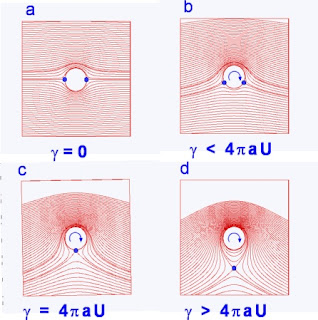## söndag 5 augusti 2012

### Unphysical Circulation TheoryThe above left picture shows potential flow around a section of a circular cylinder with increasing circulation and associated lift starting from zero at top left. Lift from circulation is supposed to explain both the Magnus effect making a top-spin tennis ball curve down and the lift of a wing according to the Kutta-Zhukovsky circulation theory.

While it is true that circulation generates lift, it is not true that lift of rotating ball or a non-rotating wing comes from circulation: It is known since long that the Magnus effect is the result of unsymmetric separation, as shown in the picture to the right, and thus not from circulation. It is known from the New Theory of Flight that the lift of a wing is generated by 3d rotational separation and not from circulation around the wing section.

To understand that the left picture depicts an unphysical flow, it is enough to see that the whole flow is redirected and bent around the cylinder, with in particular the incoming flow changing direction, as if the mere rotation of the cylinder could change the flow upstream by some unknown magical effect.

The text book explanation of lift of a wing based on the incorrect unphysical Kutta-Zhukovsky circulation theory, which has dominated for a century,  can now be replaced by a correct physical theory in accordance with observation, as required to make science.

#### 4 kommentarer:

1.I do not understand at all why you insist about the circulation losing sight of the very important aspect of the matter.
The dynamic equilibrium set out by the equation of momentum in the case of permanent state
grad(V²/2) + ω x V = - (1/ρ)grad(p)
says that the forces are only originated from grad(p) and manifest themselves as tangential acceleration grad(V²/2) and/or normal acceleration ω x V.
If the normal acceleration is missing and it is assumed that the sum of the velocities above and below the wing is twice the undisturbed velocity U, then you can calculate the lift using the circulation. In other words, the lift calculated by the circulation is the result of two approximations.
It is certainly a convenient mathematical trick for the lift calculation and this method really like the engineers as the obtained results are reliable, but it offers a partial view of the physics of lift.

It is important to realize that both Bernoulli's equation
grad(V²/2) = - (1/ρ)grad(p)
and the deflection of air (Newton's law)
ω x V = - (1/ρ)grad(p)
i.e., all the terms of the momentum equation, are essential to produce lift!

Michael

2.It 's also necessary to specify that the normal acceleration ω x V, expressed as V²/R, appears to be much more important than the tangential V²/2, because it can take any value by changing the radius of curvature R.

Michael

3.Well, you say yourself that lift by circulation is a trick, and tricks are not science.

1.Yes, but it is a trick in which you continue to give excessive importance.
Michael